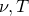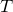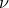## 12.7 The phy module

The phy module contains a selection of physical constants, listed in Chapter 14. It also contains the following functions:

phy.Bv()
The phy.Bv() function returns the power emitted by a blackbody of temperatureat frequencyper unit area, per unit solid angle, per unit frequency.should have physical dimensions of temperature, or be a dimensionless number, in which case it is understood to be a temperature in Kelvin.should have physical dimensions of frequency, or be a dimensionless number, in which case it is understood to be a frequency measured in Hertz. The output has physical dimensions of power per unit area per unit solid angle per unit frequency.

phy.Bvmax()
The phy.Bvmax() function returns the frequency at which the function Bv() reaches its maximum, as calculated by the Wien Displacement Law. The inputs are subject to the same constraints as above.